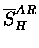Next: Absolute amplitude method Up: Corrections to in the Previous: Relative acceptance

### Combined corrections

We have looked above at the possible individual influence on the stopping fraction determination by charged particles following muon capture, decay electron energy spectra, and upstream-downstream acceptance variations. Here we combine all the factors to give a general expression. When the muons stop in M different materials, located in L different locations (such as upstream foil, downstream foil and so on), the fraction of the muon which stops in the k-th material, SARk, determined from the ratio of the amplitudes Ak' for the electron spectrum lifetime fit, is

 SkAR = (91) = (92)

where Flk is the fraction of muons stopping at the location l for the specific material k ( ), and is the effective efficiency for electron detection from muons stopped on material k at location l,

 (93)

with Qk the Huff factor for the material k, the intrinsic detector efficiency, the detector acceptance for material k at location l, and and the corrections to the efficiencies due to charged particle emission and electron energy spectrum, respectively.

In our specific case where we assume M = 2 and hydrogen is present only at the upstream foil ( ), while the muons stop in gold both at the upstream and the downstream layer with the relative fraction FAuu, FAud but nowhere else ( ), we can simplify the expression to

 (94)

whereis the ratio of the effective efficiencies for gold and hydrogen both at the upstream location, andthat for the upstream and the downstream gold:

 = (95) = (96)

The expressions above reduce to Eq. 6.11, if (i.e., gold and hydrogen have the same efficiencies for the upstream except the Huff factor), and further to Eq. 6.6 if (the same efficiencies for upstream and downstream gold) or (negligible stopping in the downstream gold).

We consider the case for counters at the sides (Ege, En1) and downstream (En2) separately. For En2, if we assume (k = Au, H and l=us, ds), and , we approximately have

 (97) (98)

On the other hand, for Ege, En1, assuming , , we have

 (99) 1, (100)

where there is some uncertainty in due to the charged particle emission effect . In addition, there is potentially a large error in the factor due to the possible non-flatness of the gold foil which could cause significant shadowing of the electrons either from gold or hydrogen. Thus, the use of the downstream counters En2, in which the shadowing effect will not be present and the charged particle emission effect is negligible, appears more reliable for the determination of the stopping fraction.

The factors in Eq. 6.15 were determined from detailed GEANT simulations  taking into account the full geometry. Simulation for the muon beam assuming a momentum of 27.0 MeV/c with of 5.7% yielded the gold stopping upstream and downstream to be Fu = 0.48 and Fd = 0.52. A separate series of simulations for the decay electrons using the energy spectrum for lead (Fig. 6.7) to represent that for gold, and the free muon to represent hydrogen gave the absolute detection efficiencies (k= H, Au; l= US, DS), presented in Table 6.11. Note that only the relative efficiencies are relevant in this context.

Table 6.11: The absolute efficiencies, (k= H, Au; l= US, DS) for electron detection, calculated by GEANT simulations . Uncertainties given are statistical only.
 Efficiency US DS H Au Au Ege 6.03(8) 4.88(7) 4.90(7) En1 6.14(8) 5.02(7) 4.93(7) En2 5.11(7) 4.06(7) 5.59(7)

Table 6.12: The correction factors,in Eq. 6.15, and assumed quantities for the derivation by Eqs. 6.166.17. The first row is the average of Ege and En1. Values with `*' are taken from the ratio of the efficiencies calculated by a GEANT simulation given in Table 6.11.
 Detector QAuEgeEn1 0.85 0.813* 1.04 1 0.719 1.007* En2 0.85 0.795* 1 1 0.676 1.375*

Table 6.13: The uncorrected stopping fractionfrom Eq. 6.6 and the corrected one SHAR from Eq.6.15 for the runs 1650, 1654. The errors on SHAR for Ege, En1 include estimated 4% (relative) uncertainties due to the charge particle emission. The other errors are statistical only.
 Detector(%) SHAR (%) Ege 26.6 (4) 23.5 (12) En1 26.5 (4) 23.5 (12) En2 24.3 (4) 23.4 (7)

The correction factors, which take into account the differences in solid angle for upstream and downstream, electron energy spectra between gold and hydrogen, and effects of charged particle emission, can now be determined. Together with the assumed quantities, the values ofandare given in Table 6.12. Note that assuming ,is the direct ratio of the electron detection efficiencies . As mentioned before, can have relatively large uncertainties, due to possible errors in and .

The effect of these corrections on the stopping fraction is summarized in Table 6.13. The uncertainty in the estimated value of (1.04) for Ege, En1 is assumed to be 0.04, which is included quadratically in the uncertainties presented in the table. Possible errors in for Ege and En1 are not included. The error for En2 is statistical only.

The total of about -12% relative correction to for Ege, En1, is dominated by the -14% correction due to the difference in the relative electron detection efficiency (which in turn is dominated presumably by the electron energy spectrum effect), partly offset by a +3% effect due to charged particle emission.

For En2, the correction to is rather small as a result of cancellation between the -16% relative correction, due to the difference in the energy spectrum, and the +14% correction, due to the difference in the upstream-downstream relative acceptance. The latter correction in is reasonably close to the approximate +10% given on page, estimated with simplified assumptions without detailed simulations.Next: Absolute amplitude method Up: Corrections to in the Previous: Relative acceptance